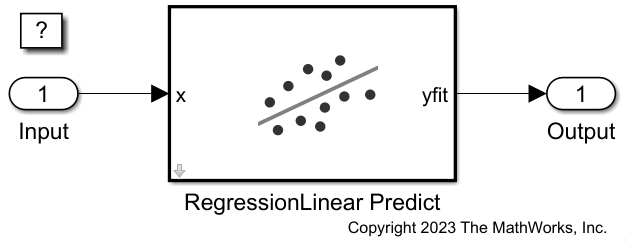# Predict Responses Using RegressionLinear Predict Block

Since R2023a

This example shows how to use the RegressionLinear Predict block for response prediction in Simulink®. The block accepts an observation (predictor data) and returns the predicted response for the observation using the trained regression linear model.

### Train Regression Model

Simulate 10,000 observations from this model

`$y={x}_{100}+2{x}_{200}+e.$`

• $X={x}_{1},...,{x}_{1000}$ is a 10,000-by-1000 sparse matrix with 10% nonzero standard normal elements.

• e is a random normal error with mean 0 and standard deviation 0.3.

```rng("default") % For reproducibility n = 1e4; d = 1e3; nz = 0.1; X = sprandn(n,d,nz); Y = X(:,100) + 2*X(:,200) + 0.3*randn(n,1);```

Suppose that 90% of the data is observed, and the rest is unseen. Partition the data into present and future samples.

```c = cvpartition(n,Holdout=0.1); idxPrsnt = training(c); idxFtr = test(c); prsntX = X(idxPrsnt,:); prsntY = Y(idxPrsnt); ftrX = X(idxFtr,:); ftrY = Y(idxFtr);```

Train a regression linear model.

`linearMdl = fitrlinear(prsntX,prsntY);`

`linearMdl` is a `RegressionLinear` model.

This example provides the Simulink model `slexRegressionLinearPredictExample.slx`, which includes the RegressionLinear Predict block. You can open the Simulink model or create a new model as described in this section.

Open the Simulink model `slexRegressionLinearPredictExample.slx`.

```SimMdlName = "slexRegressionLinearPredictExample"; open_system(SimMdlName)```If you open the Simulink model, then the software runs the code in the `PreLoadFcn` callback function before loading the Simulink model. The `PreLoadFcn` callback function of `slexRegressionLinearPredictExample` includes code to check if your workspace contains the `linearMdl` variable for the trained model. If the workspace does not contain the variable, `PreLoadFcn` loads the sample data, trains the linear model, and creates an input signal for the Simulink model. To view the callback function, in the Setup section on the Modeling tab, click Model Settings and select Model Properties. Then, on the Callbacks tab, select the `PreLoadFcn` callback function in the Model callbacks pane.

To create a new Simulink model, open the Blank Model template and add the RegressionLinear Predict block. Add the Inport and Outport blocks and connect them to the RegressionLinear Predict block.

Double-click the RegressionLinear Predict block to open the Block Parameters dialog box. Specify the name of the workspace variable that contains the trained linear model. The default variable name is `linearMdl`. Click the Refresh button. The dialog box displays the options used to train the linear model `linearMdl` under Trained Machine Learning Model.Create an input signal in the form of a structure array for the Simulink model. The structure array must contain these fields:

• `time` — The points in time at which the observations enter the model. In this example, the duration includes the integers from 0 through `nftrX - 1`, where `nftrX` is the number of samples in the input data. The orientation must correspond to the observations in the predictor data. So, in this case, `time` must be a column vector.

• `signals` — A 1-by-1 structure array describing the input data and containing the fields `values` and `dimensions`, where `values` is a matrix of predictor data, and `dimensions` is the number of predictor variables.

Create an appropriate structure array for the future samples in the sparse matrix `ftrX` after converting the matrix to full storage organization by using the `full` function.

```[nftrX,p] = size(ftrX); inputStruct.time = (1:nftrX)' - 1; inputStruct.signals(1).values = full(ftrX); inputStruct.signals(1).dimensions = p;```

To import signal data from the workspace:

• Open the Configuration Parameters dialog box. On the Modeling tab, click Model Settings.

• In the Data Import/Export pane, select the Input check box and enter `inputStruct` in the adjacent text box.

• In the Solver pane, under Simulation time, set Stop time to `inputStruct.time(end)`. Under Solver selection, set Type to `Fixed-step`, and set Solver to `discrete (no continuous states)`. Under Solver details, set `Fixed-step size` to 1. These settings enable the model to run the simulation for each sample in `inputStruct`.

`sim(SimMdlName);`